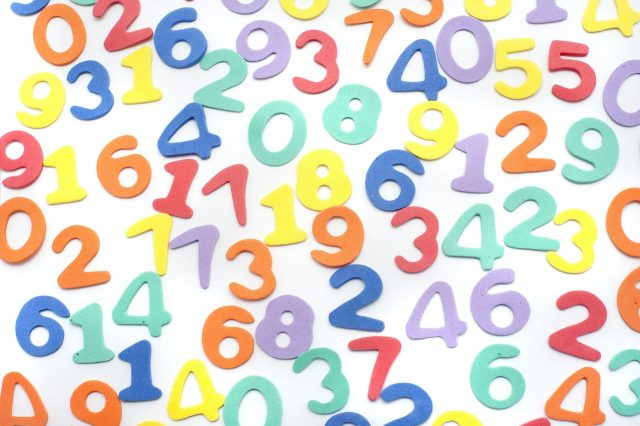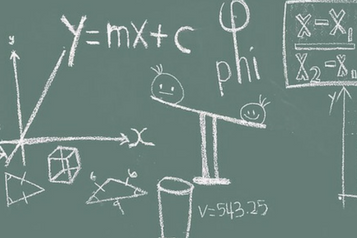Math Program

Little Mountain Learning Academy is excited to announce our new in­-house developed math curriculum for students to meet and exceed the BC Provincial Learning Outcomes within the subject. Our math program covers grades 3 to 7, and should be considered by those students who wish to move ahead of their current grade, or by those students who need extra math support. The math program allows students to work through varying levels, and tests at their own speed as courses are completed independently with the aid of a specialized math teacher.Learning ObjectivesIn the first two levels of the math program, the students will focus on building a solid foundation. The students will build their math vocabulary and utilize those terms in different word problems as well. Addition, subtraction, multiplication, division, and fractions will be covered in these two levels. Variables and equations are introduced in our level 4 program.Students in the intermediate levels understand and have acquired the skills to perform the basic operations. Decimals and fractions will be studied in depth at these levels. In level 5, the students will be introduced to the Cartesian coordinate and coordinate planes. Students will learn about ratios, integers, statistics, probability, and algebra.Students that advance to our level 7 program have a good understanding of concepts learned in the intermediate levels. A number of new concepts are introduced at level 7. Students will learn how to add and subtract fractions. They will also work with linear equations. In this level, students will continue to develop a better understanding of the preservation of equality, different expressions, and equations.(Tap to view teacher bio)